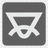The Angles of a Triangle
Elementary+
English FR JA RU UK

You are given the lengths for each side on a triangle. You need to find all three angles for this triangle. If the given side lengths cannot form a triangle (or form a degenerated triangle), then you must return all angles as 0 (zero). The angles should be represented as a list of integers in ascending order. Each angle is measured in degrees and rounded to the nearest integer number (Standard mathematical rounding).Input: The lengths of the sides of a triangle as integers.

Output: Angles of a triangle in degrees as sorted list of integers.

Example:

```checkio(4, 4, 4) == [60, 60, 60]
checkio(3, 4, 5) == [37, 53, 90]
checkio(2, 2, 5) == [0, 0, 0]```

How it is used: This is a classical geometric task. The ideas can be useful in topography and architecture. With this concept you can measure an angle without the need for a protractor.

Precondition:
0 < a,b,c ≤ 1000